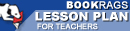Lesson Plans
Related Topics

# Daily Lessons for Teaching Impulse

This set of Lesson Plans consists of approximately 119 pages of tests, essay questions, lessons, and other teaching materials.
 View a FREE sample## Lesson 1 (from Chapter 1-6, Conner to Vanessa)

### Objective

Every story begins with an introduction where the tone, characters and plot of the story are introduced. The introduction serves to whet the appetite of the reader and hook them on the story, thus making them want to read further. The objective of this lesson is to examine the introduction and set-up of the novel.

### Lesson

Class Discussion: Imagine a general plot line. Where is the introduction of a story on that line? What is the purpose of an introduction? Do all stories have one? How does the introduction serve the reader? What happens when a story has a poor or uninteresting introduction?

Individual Assignment: Draw a plot line. Make sure sections are labeled for Introduction, Rising Action, Climax, Falling Action and Resolution. Under the Introduction segment, write in 2 -3 specific incidents from the beginning of the book that you found to be compelling. Save this...

 This section contains 7,488 words (approx. 25 pages at 300 words per page) View a FREE sample